Function Repository Resource:

# InverseGrayCode

Find the integer corresponding to a given Gray code

Contributed by: Jan Mangaldan
 ResourceFunction["InverseGrayCode"][{b1,b2,…}] gives the integer corresponding to the Gray code represented by the bits bi.

## Examples

### Basic Examples (1)

Find the integer corresponding to a given Gray code:

 In:=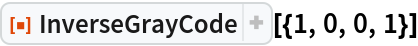Out=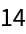### Applications (2)

Use InverseGrayCode to generate the Thue–Morse sequence:

 In:=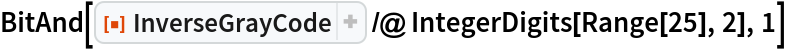Out=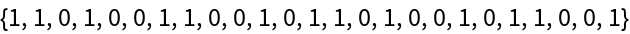Compare with the built-in ThueMorse:

 In:=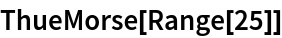Out=Use InverseGrayCode to generate the Rudin–Shapiro sequence:

 In:=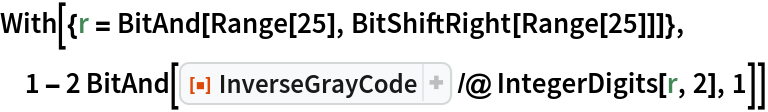Out=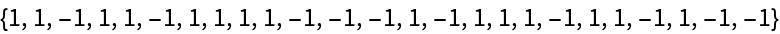Compare with the built-in RudinShapiro:

 In:=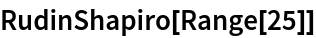Out=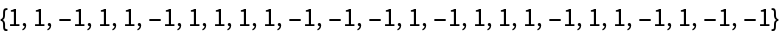### Properties and Relations (1)

InverseGrayCode is the inverse of the resource function GrayCode:

 In:=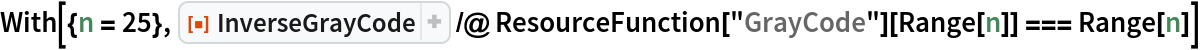Out=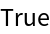## Version History

• 1.0.0 – 28 June 2021